# Statistics Confidence Intervals Confidence Intervals Confidence Intervals Confidence

• Slides: 80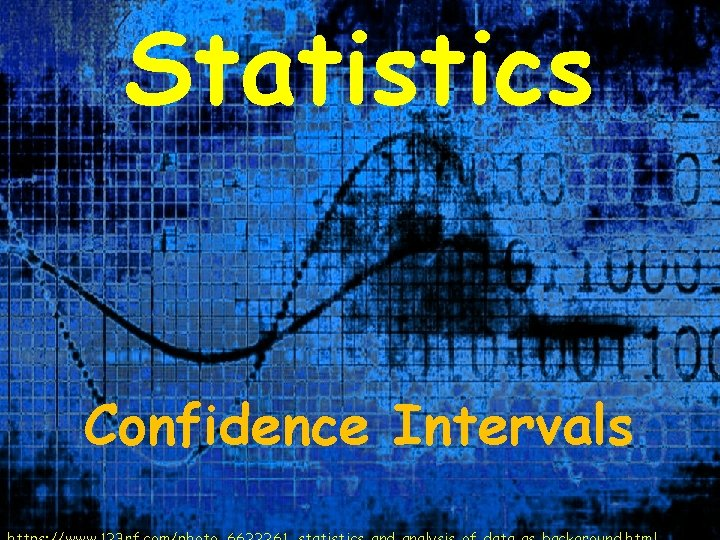Statistics Confidence IntervalsConfidence Intervals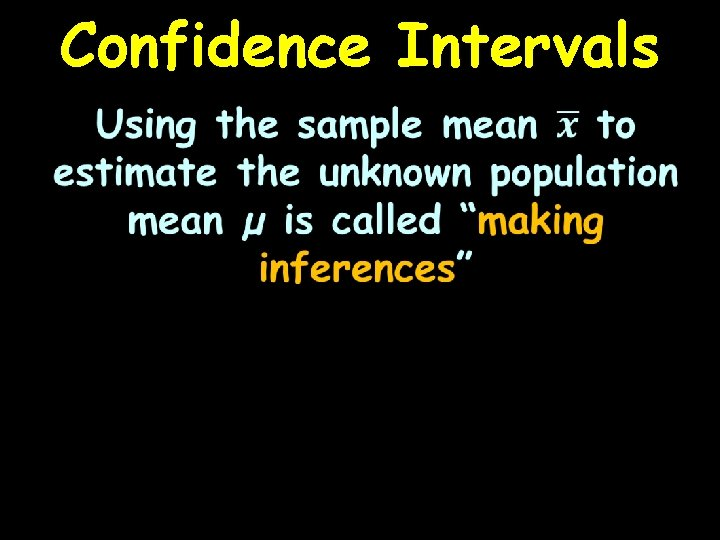Confidence IntervalsConfidence Intervals If you can assume the distribution of the sample means is normal, you can use the normal distribution probabilities for making probability statements about µ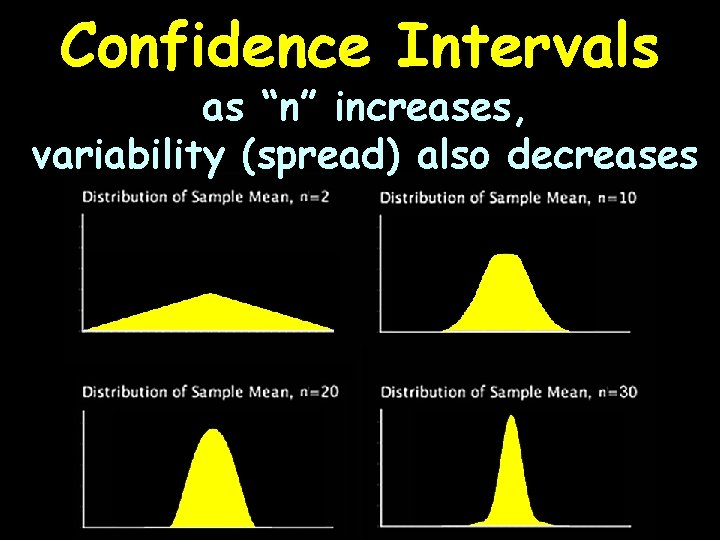Confidence Intervals as “n” increases, variability (spread) also decreases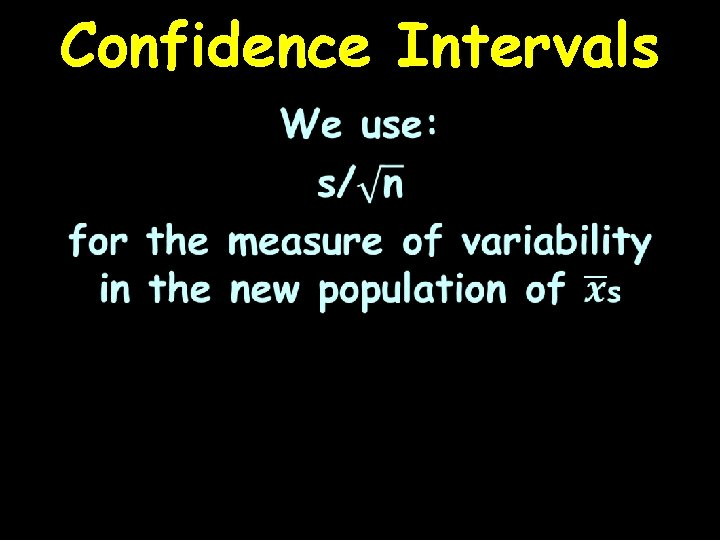Confidence IntervalsConfidence IntervalsConfidence Intervals So our normal curve for the true value of the population mean µ is: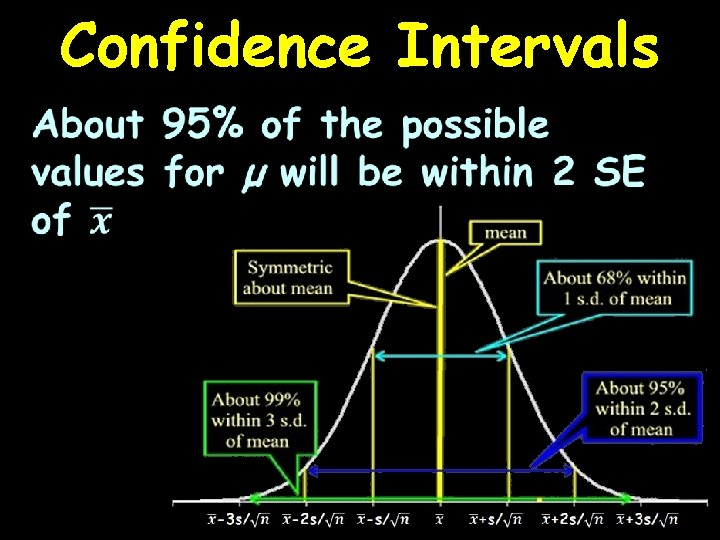Confidence Intervals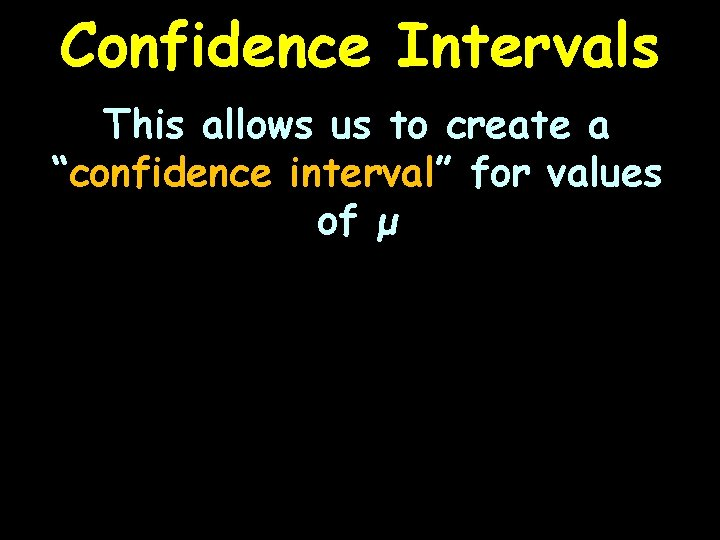Confidence Intervals This allows us to create a “confidence interval” for values of μ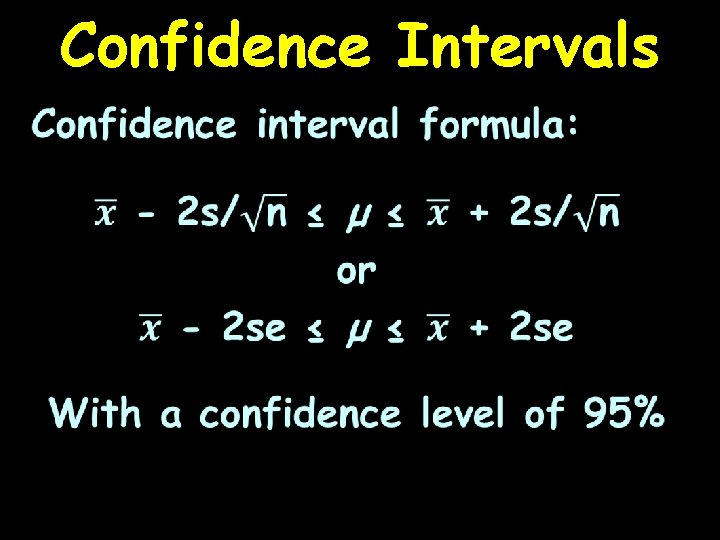Confidence Intervals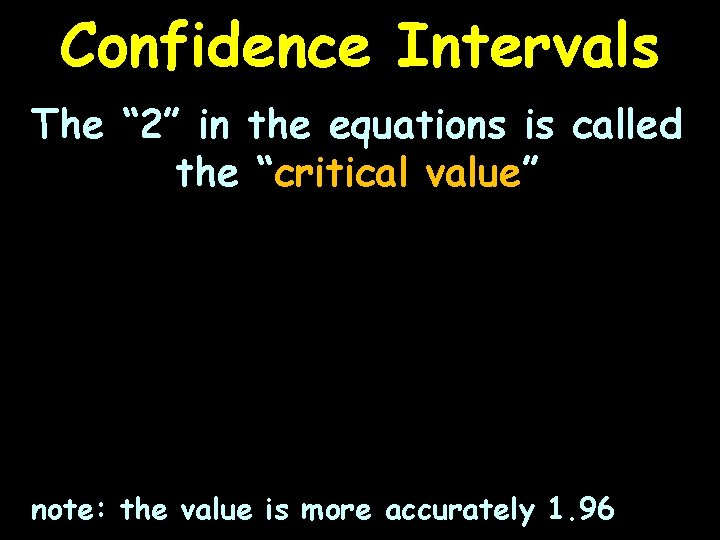Confidence Intervals The “ 2” in the equations is called the “critical value” note: the value is more accurately 1. 96Confidence Intervals It comes from the normal curve, which gives us the 95%Confidence IntervalsCONFIDENCE INTERVALS PROJECT QUESTION What if we wanted a confidence level of 99%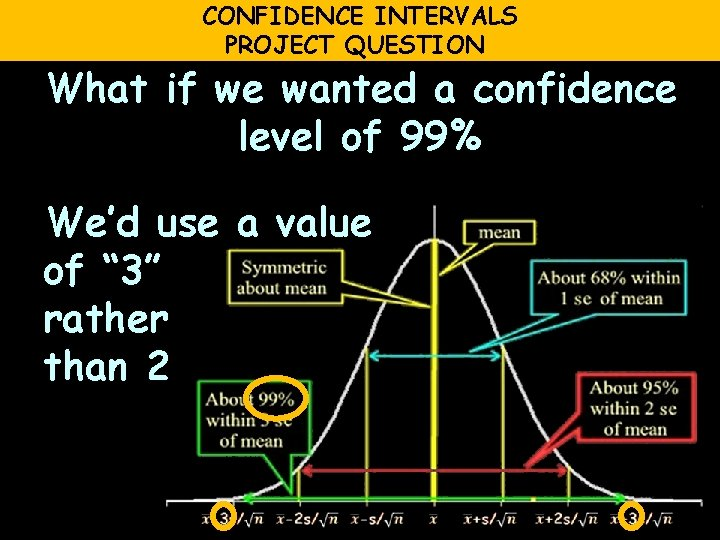CONFIDENCE INTERVALS PROJECT QUESTION What if we wanted a confidence level of 99% We’d use a value of “ 3” rather than 2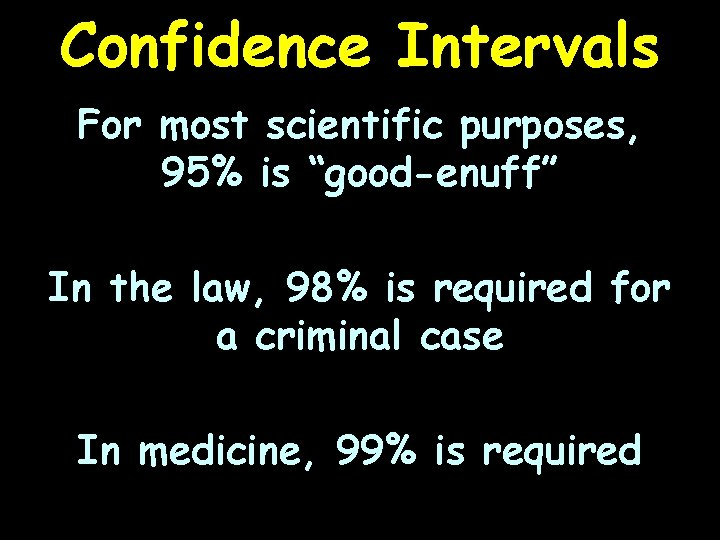Confidence Intervals For most scientific purposes, 95% is “good-enuff” In the law, 98% is required for a criminal case In medicine, 99% is required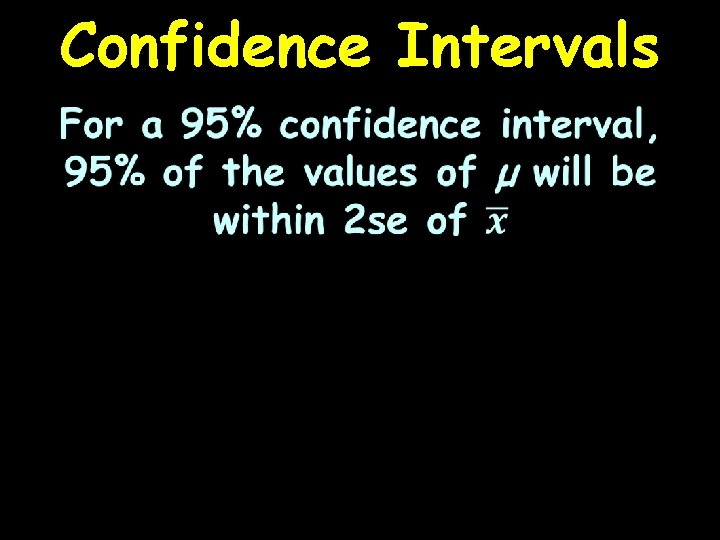Confidence IntervalsConfidence Intervals If we use the confidence interval to estimate a likely range for true values of μ, we will be right 95% of the time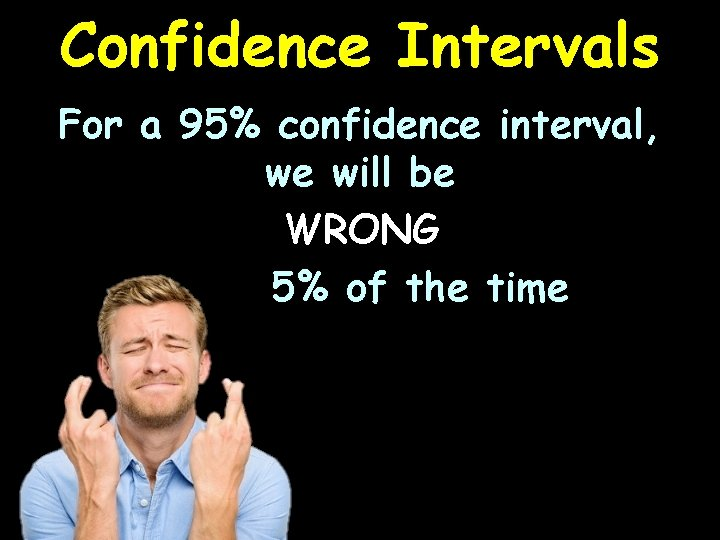Confidence Intervals For a 95% confidence interval, we will be WRONG 5% of the time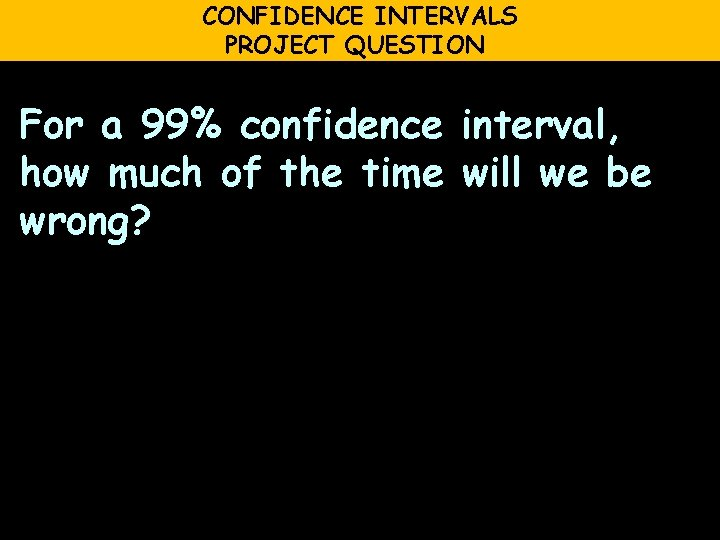CONFIDENCE INTERVALS PROJECT QUESTION For a 99% confidence interval, how much of the time will we be wrong?CONFIDENCE INTERVALS PROJECT QUESTION For a 99% confidence interval, how much of the time will we be wrong? we will be wrong 1% of the timeConfidence Intervals The percent of time we are willing to be wrong is called “α” (“alpha”) or “the α-level”Confidence Intervals Everyday use of confidence intervals: You will frequently hear that a poll has a candidate ahead by 10 points with a margin of error of 3 pointsConfidence Intervals This means: 10 -3 ≤ true difference ≤ 10+3 Or, the true difference is between 7 and 13 points (with 95% likelihood)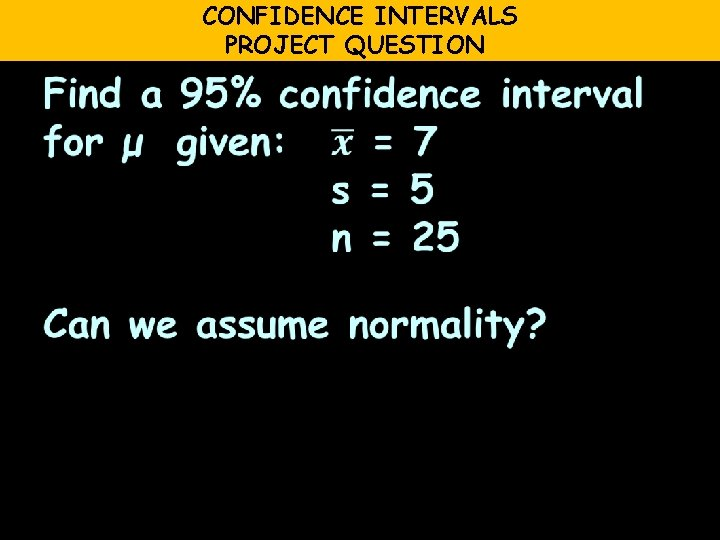CONFIDENCE INTERVALS PROJECT QUESTION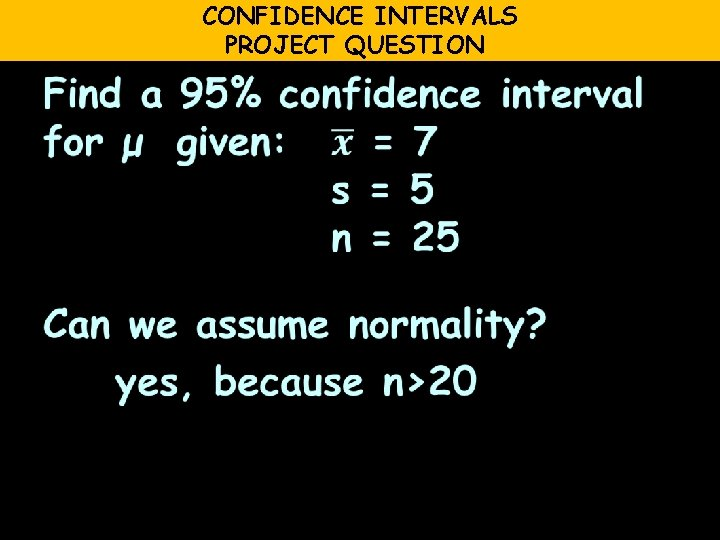CONFIDENCE INTERVALS PROJECT QUESTION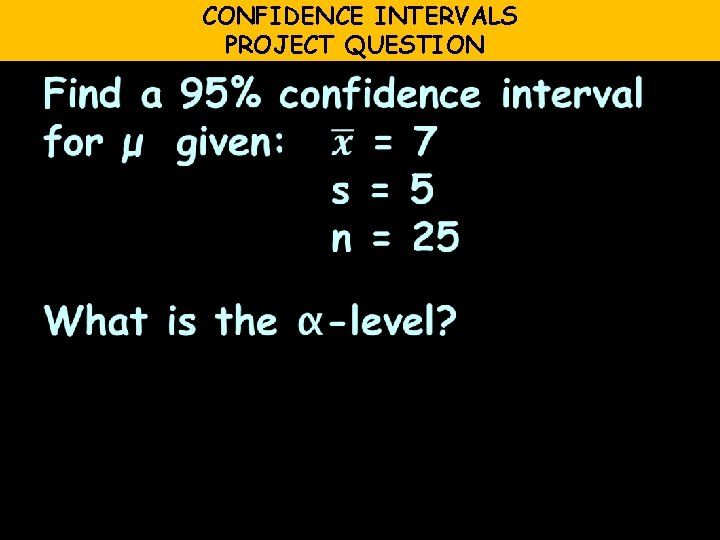CONFIDENCE INTERVALS PROJECT QUESTION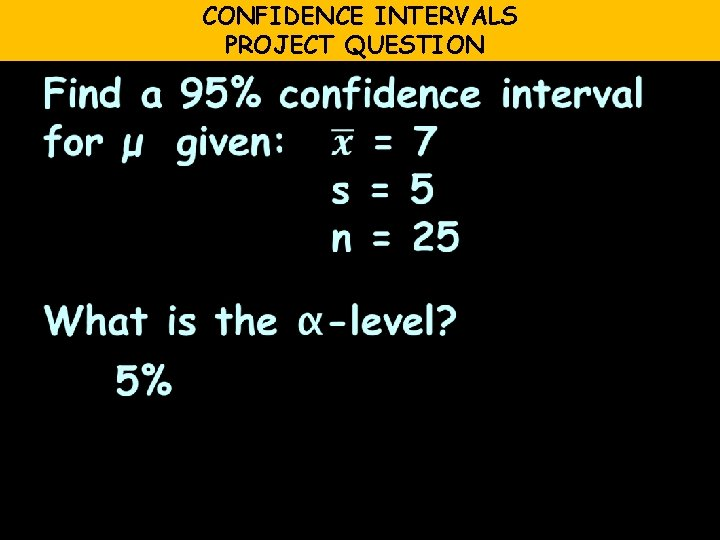CONFIDENCE INTERVALS PROJECT QUESTIONCONFIDENCE INTERVALS PROJECT QUESTIONCONFIDENCE INTERVALS PROJECT QUESTIONCONFIDENCE INTERVALS PROJECT QUESTIONCONFIDENCE INTERVALS PROJECT QUESTION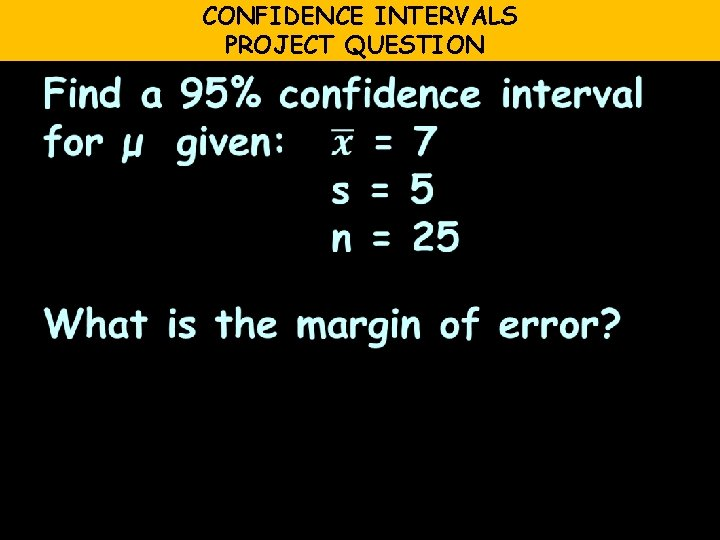CONFIDENCE INTERVALS PROJECT QUESTIONCONFIDENCE INTERVALS PROJECT QUESTION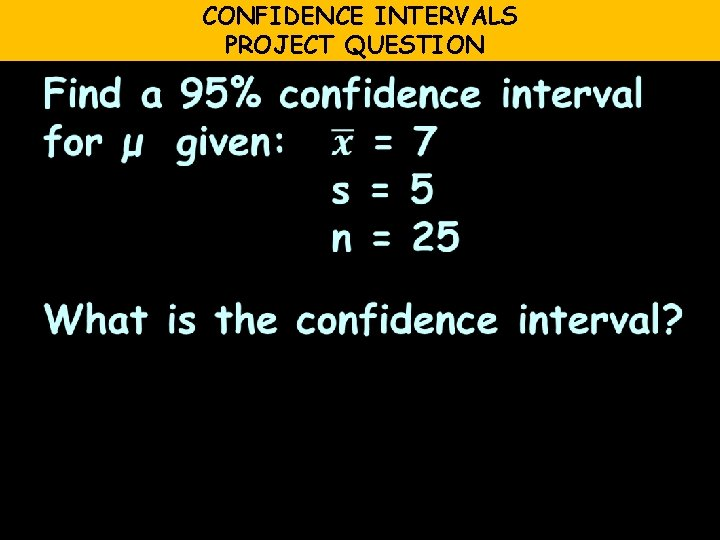CONFIDENCE INTERVALS PROJECT QUESTION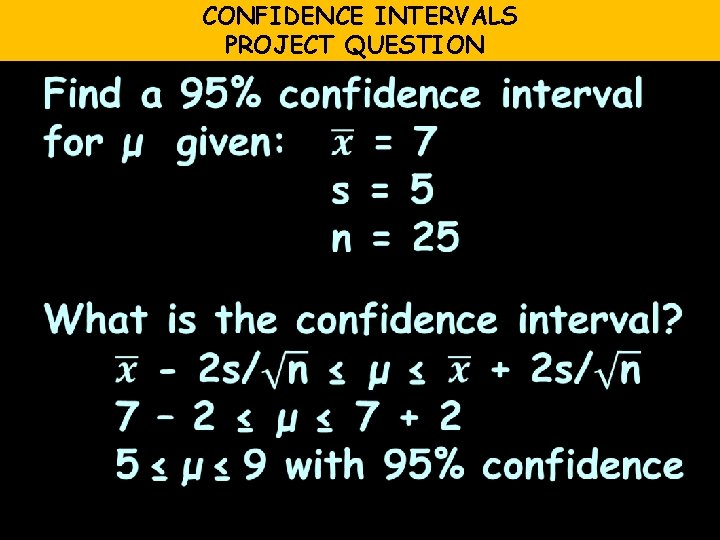CONFIDENCE INTERVALS PROJECT QUESTION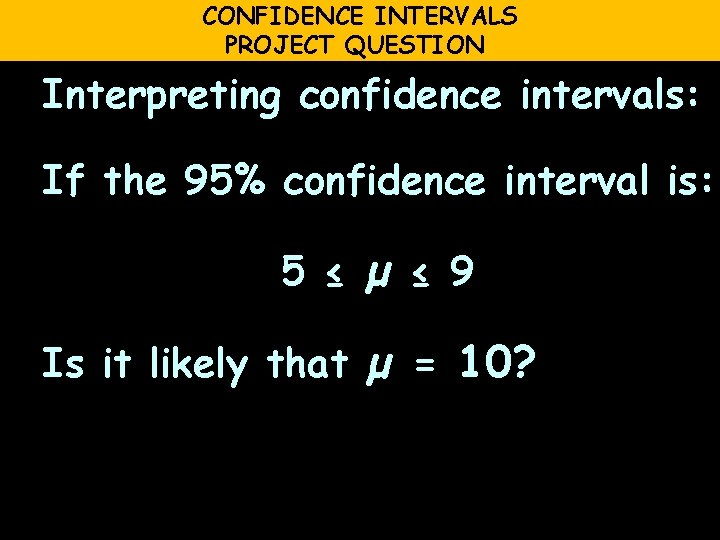CONFIDENCE INTERVALS PROJECT QUESTION Interpreting confidence intervals: If the 95% confidence interval is: 5 ≤ µ ≤ 9 Is it likely that µ = 10?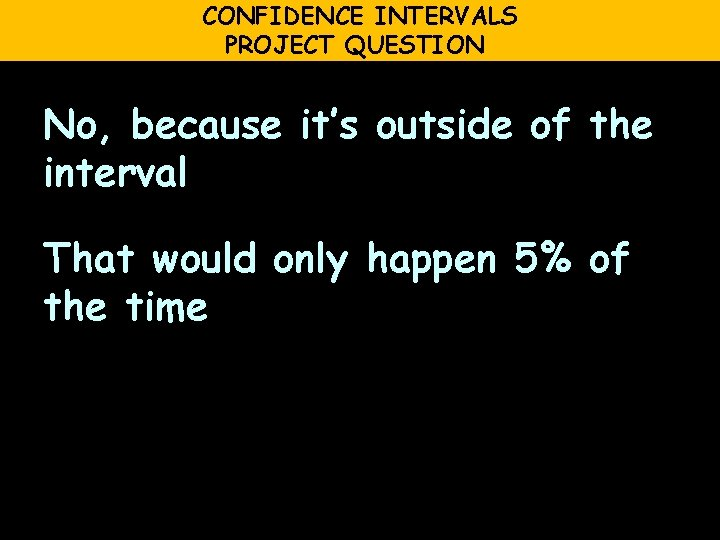CONFIDENCE INTERVALS PROJECT QUESTION No, because it’s outside of the interval That would only happen 5% of the timeConfidence Intervals PROJECT QUESTIONConfidence Intervals PROJECT QUESTIONConfidence Intervals PROJECT QUESTIONConfidence Intervals PROJECT QUESTION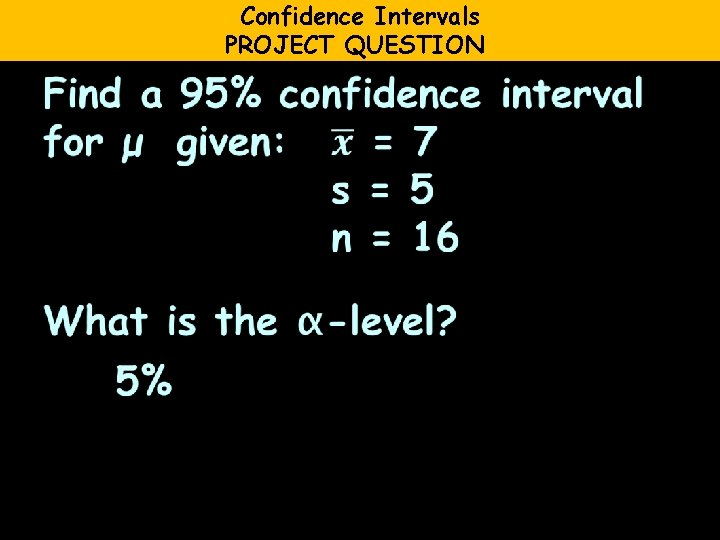Confidence Intervals PROJECT QUESTION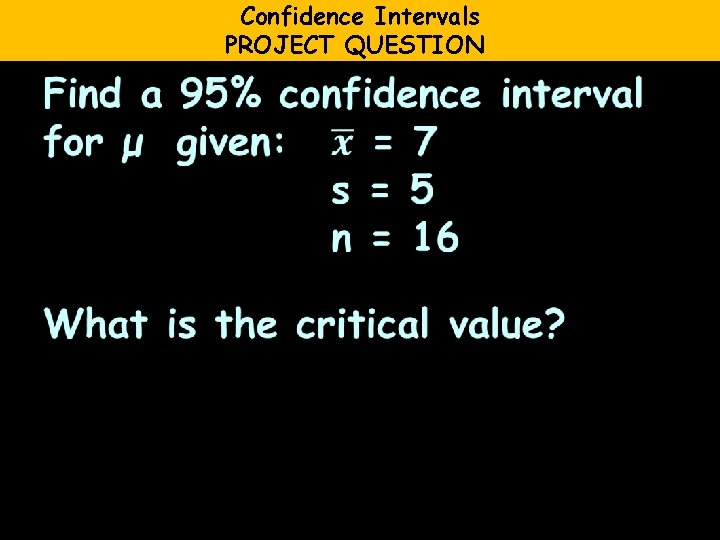Confidence Intervals PROJECT QUESTION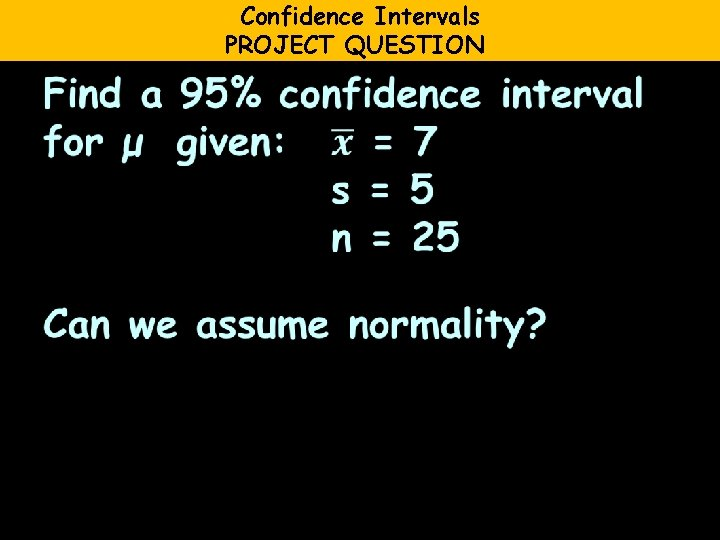Confidence Intervals PROJECT QUESTION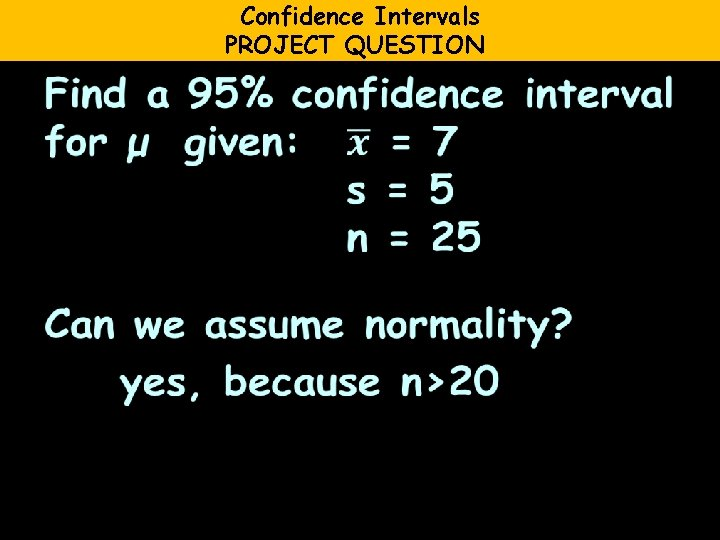Confidence Intervals PROJECT QUESTIONConfidence Intervals PROJECT QUESTIONConfidence Intervals PROJECT QUESTIONConfidence Intervals PROJECT QUESTIONConfidence Intervals PROJECT QUESTION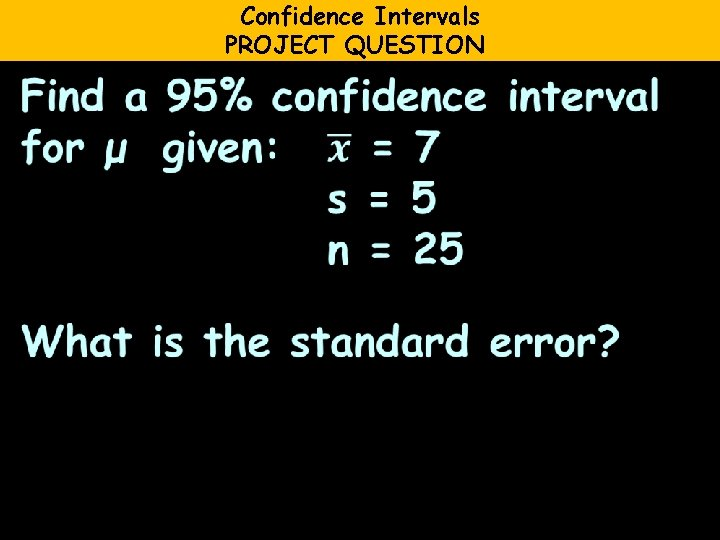Confidence Intervals PROJECT QUESTION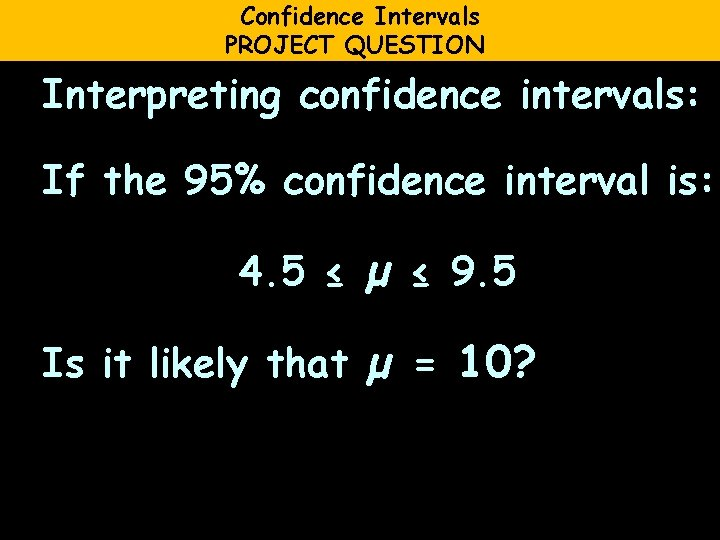Confidence Intervals PROJECT QUESTION Interpreting confidence intervals: If the 95% confidence interval is: 4. 5 ≤ µ ≤ 9. 5 Is it likely that µ = 10?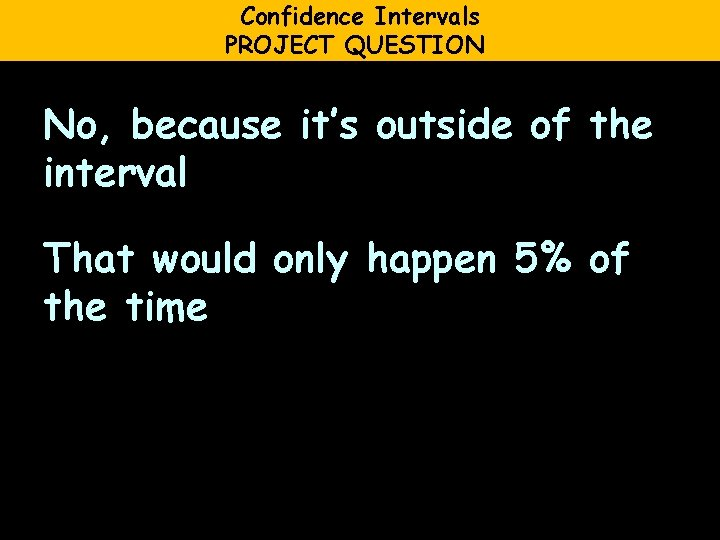Confidence Intervals PROJECT QUESTION No, because it’s outside of the interval That would only happen 5% of the timeCONFIDENCE INTERVALS PROJECT QUESTION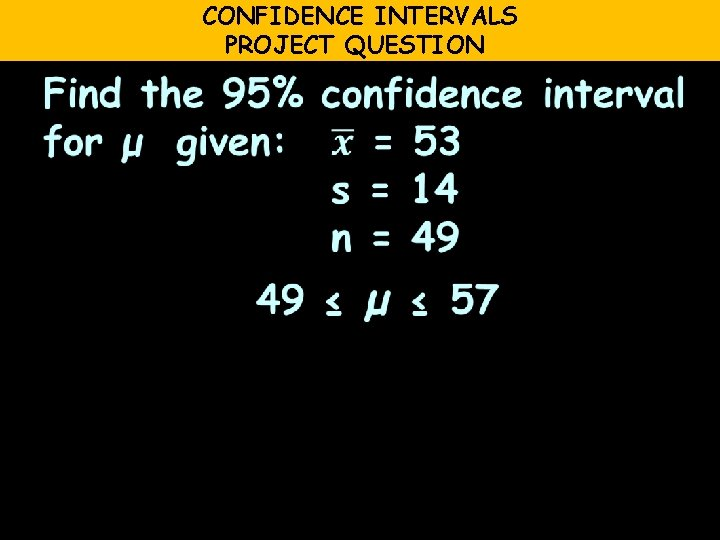CONFIDENCE INTERVALS PROJECT QUESTION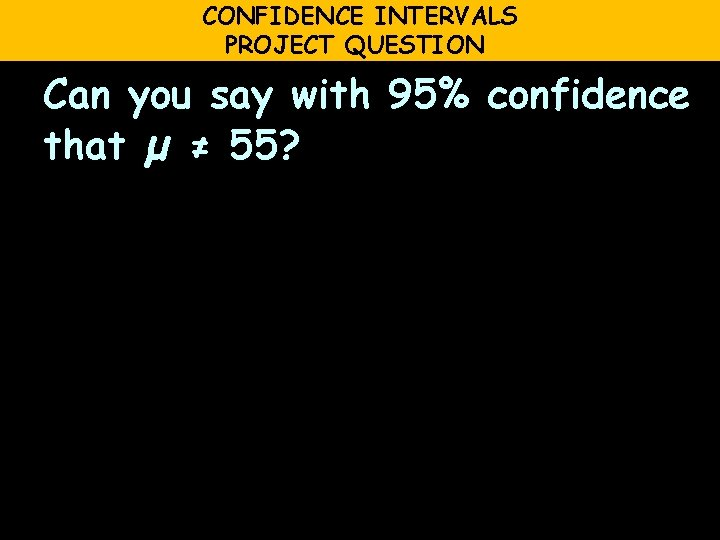CONFIDENCE INTERVALS PROJECT QUESTION Can you say with 95% confidence that µ ≠ 55?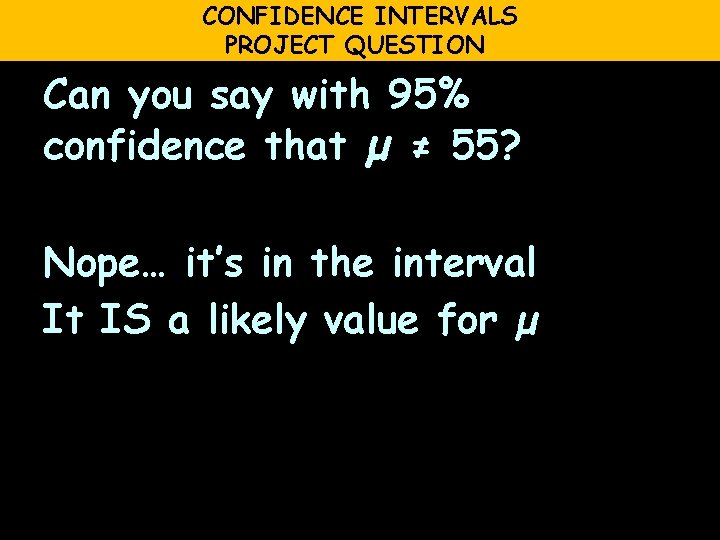CONFIDENCE INTERVALS PROJECT QUESTION Can you say with 95% confidence that µ ≠ 55? Nope… it’s in the interval It IS a likely value for µCONFIDENCE INTERVALS PROJECT QUESTION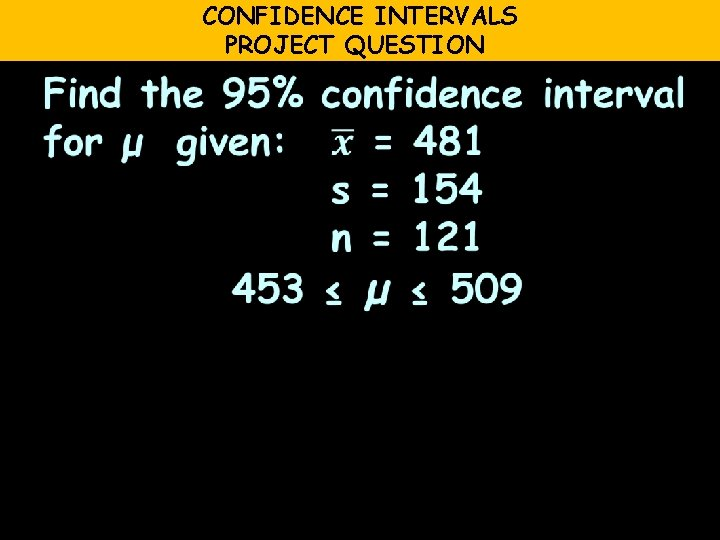CONFIDENCE INTERVALS PROJECT QUESTION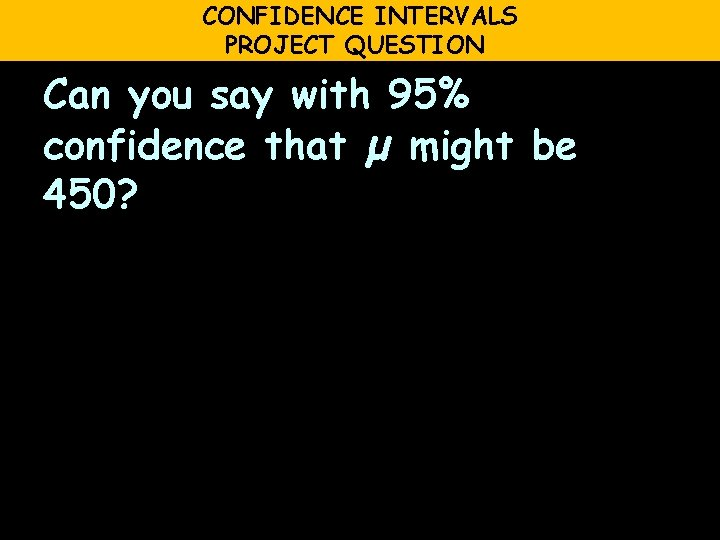CONFIDENCE INTERVALS PROJECT QUESTION Can you say with 95% confidence that µ might be 450?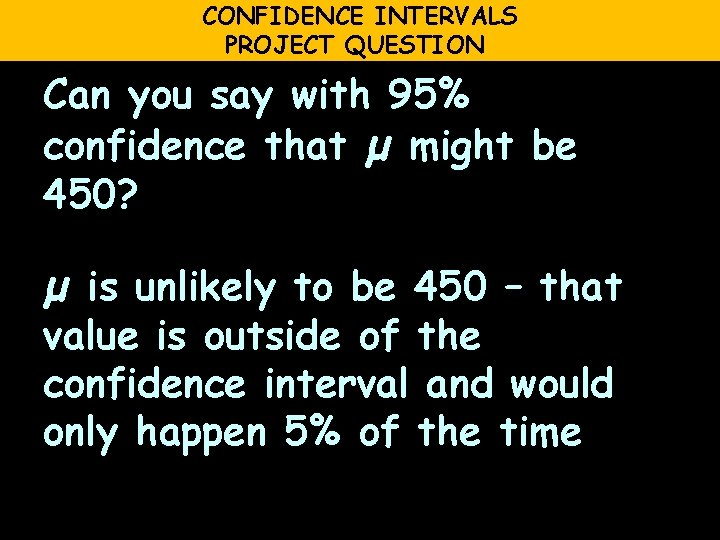CONFIDENCE INTERVALS PROJECT QUESTION Can you say with 95% confidence that µ might be 450? µ is unlikely to be 450 – that value is outside of the confidence interval and would only happen 5% of the timeConfidence Intervals You will have a smaller interval if you have a larger value for nConfidence Intervals So you want to take the LARGEST sample you can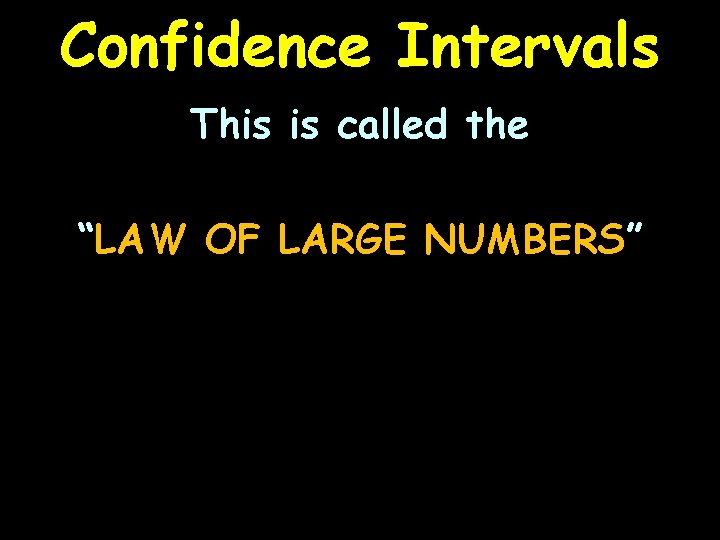Confidence Intervals This is called the “LAW OF LARGE NUMBERS”Confidence Intervals What if you have a sample size smaller than 20? ? ?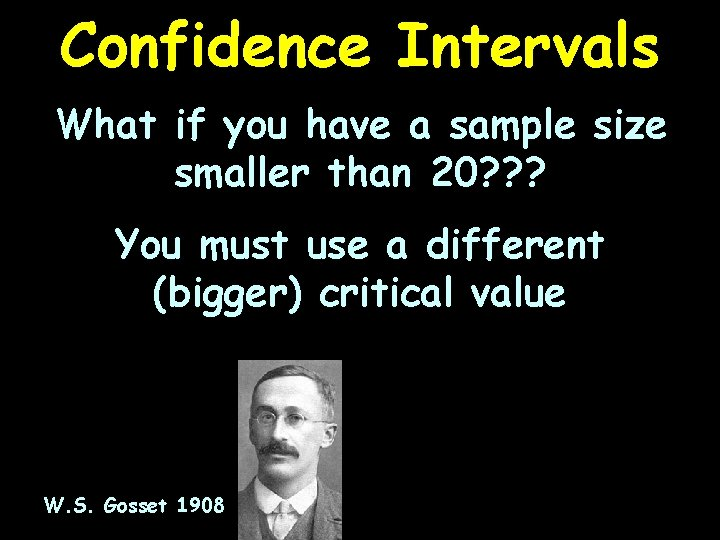Confidence Intervals What if you have a sample size smaller than 20? ? ? You must use a different (bigger) critical value W. S. Gosset 1908Questions?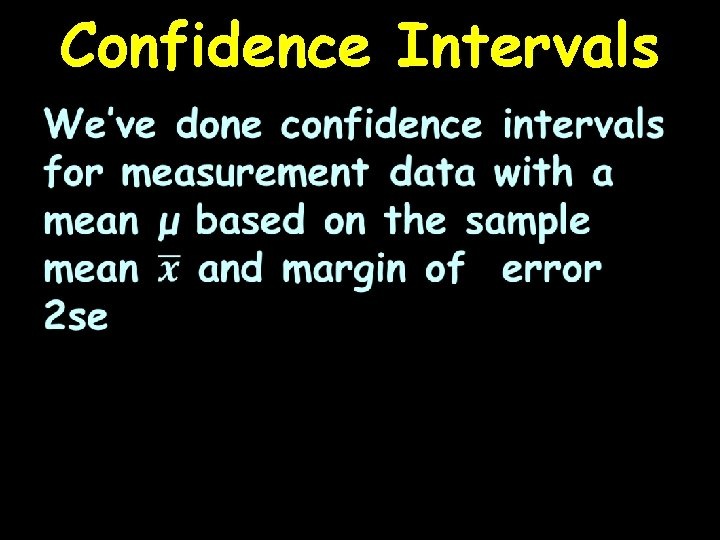Confidence IntervalsCI for Proportions What about count data and proportions?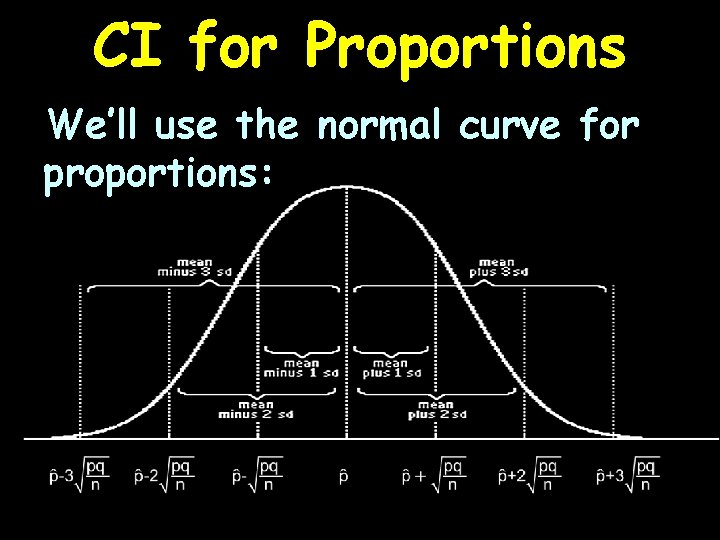CI for Proportions We’ll use the normal curve for proportions:CI FOR PROPORTIONS PROJECT QUESTIONCI FOR PROPORTIONS PROJECT QUESTIONCI FOR PROPORTIONS PROJECT QUESTIONCI FOR PROPORTIONS PROJECT QUESTIONCI FOR PROPORTIONS PROJECT QUESTIONCI FOR PROPORTIONS PROJECT QUESTIONCI FOR PROPORTIONS PROJECT QUESTIONQuestions?